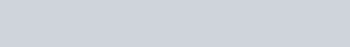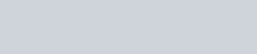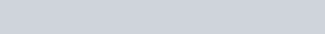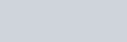Probability – JEE Advanced Previous Year Questions with Solutions
JEE Advanced Previous Year Questions of Math with Solutions are available at eSaral. Practicing JEE Advanced Previous Year Papers Questions of mathematics will help the JEE aspirants in realizing the question pattern as well as help in analyzing weak & strong areas.eSaral helps the students in clearing and understanding each topic in a better way. eSaral also provides complete chapter-wise notes of Class 11th and 12th both for all subjects.Besides this, eSaral also offers NCERT Solutions, Previous year questions for JEE Main and Advance, Practice questions, Test Series for JEE Main, JEE Advanced and NEET, Important questions of Physics, Chemistry, Math, and Biology and many more.Download
Q. A fair die is tossed repeatedly until a six is obtained. Let X denote thenumber of tosses required.(A) The probability that X = 3 equals –(A) $\frac{25}{216}$(B) $\frac{25}{36}$(C) $\frac{5}{36}$(D) $\frac{125}{216}$(B) The probability that $\mathrm{X} \geq 3$ equals –(A) $\frac{125}{216}$(B) $\frac{25}{36}$(C) $\frac{5}{36}$(D) $\frac{25}{216}$(C) The conditional probability that X  6 given X > 3 equals –(A) $\frac{125}{216}$(B) $\frac{25}{216}$(C) $\frac{5}{36}$(D) $\frac{25}{36}$ [JEE 2009, 4+4+4]

Download eSaral App for Video Lectures, Complete Revision, Study Material and much more...

Sol. ( (a) A (b) B (c) D ) (A) $\mathrm{P}(\mathrm{X}=3)=\left(\frac{5}{6}\right)\left(\frac{5}{6}\right)\left(\frac{1}{3}\right)=\frac{25}{216}$(B) $P(X \leq 2)=\frac{1}{6}+\frac{5}{6} \times \frac{1}{6}=\frac{11}{36}$(C) For $\mathrm{X} \geq 6$ the probability is $\begin{array}{l}{\frac{5^{5}}{6^{6}}+\frac{5^{5}}{6^{7}}+\ldots \ldots+\infty=\frac{5^{5}}{6^{6}}\left(\frac{1}{1-5 / 6}\right)=\left(\frac{5}{6}\right)^{5}} \\ {\text { For } \mathrm{X}>3} \\{\frac{5^{3}}{6^{4}}+\frac{5^{4}}{6^{5}}+\frac{5^{5}}{6^{6}}+\ldots \ldots \infty=\left(\frac{5}{6}\right)^{3}} \end{array}$Hence the conditional probability is $\frac{(5 / 6)^{6}}{(5 / 6)^{3}}=\frac{25}{36}$

Q. (A) Let $\omega$ be a complex cube root of unity with $\omega \neq 1 .$ A fair die is thrown three times. If $\mathrm{r}_{1}, \mathrm{r}_{2}$ and $\mathrm{r}_{3}$ are the numbers obtained on the die, then the probability that $\omega^{\mathrm{r}_{1}}+\omega^{\mathrm{r}_{2}}+\omega^{\mathrm{rs}}=0$ is –(A) $\frac{1}{18}$(B) $\frac{1}{9}$(C) $\frac{2}{9}$(D) $\frac{1}{36}$(B) A signal which can be green or red with probability $\frac{4}{5}$ and $\frac{1}{5}$ respectively, is received by station $A$ and then transmitted to station B. The probability of each station receiving the signal correctly is $\frac{3}{4} .$ If the signal received at station $\mathrm{B}$ is green, then the probability that the original signal was green is –(A) $\frac{3}{5}$(B) $\frac{6}{7}$(C) $\frac{20}{23}$(D) $\frac{9}{20}$ [JEE 2010, 3+5]

Download eSaral App for Video Lectures, Complete Revision, Study Material and much more...

Sol. ( (a) C (b) C )(A) $\mathrm{r}_{1}, \mathrm{r}_{2}, \mathrm{r}_{3}$ can be from the set $(3,6),(1,4)$ or $(2,5)$ which can be done in $2 \times 2 \times 2=$8 ways and these can be arranged in $3 !$ ways$\text { Probability }=\frac{3 ! \times 8}{216}=\frac{2}{9}$(B) $\mathrm{C}:$ Correct signal is transmitted$\overline{\mathrm{C}}:$ false signal is transmitted$\mathrm{G}:$ Original signal is green$\mathrm{R}:$ Original signal is red$\mathrm{K}:$ Signal received at station $\mathrm{B}$ is green. $\mathrm{P}(\mathrm{G} / \mathrm{K})=\frac{\mathrm{P}(\mathrm{G}) \cdot \mathrm{P}(\mathrm{K} / \mathrm{G})}{\mathrm{P}(\mathrm{K})}$$\mathrm{P}(\mathrm{G} / \mathrm{K})=\frac{\mathrm{P}(\mathrm{G}) \cdot \mathrm{P}(\mathrm{K} / \mathrm{G})}{\mathrm{P}(\mathrm{K})}$$=\frac{\mathrm{P}(\mathrm{GCC})+\mathrm{P}(\mathrm{GC} \overline{\mathrm{C}})}{\mathrm{P}(\mathrm{GCC})+\mathrm{P}(\mathrm{G} \overline{\mathrm{C}} \overline{\mathrm{C}})+\mathrm{P}(\mathrm{RC} \overline{\mathrm{C}})+\mathrm{P}(\mathrm{R} \overline{\mathrm{C}} \mathrm{C})}$$=\frac{\frac{4}{5} \times \frac{3}{4} \times \frac{3}{4}+\frac{4}{5} \times \frac{1}{4} \times \frac{1}{4}}{\frac{4}{5} \times \frac{3}{4} \times \frac{3}{4}+\frac{4}{5} \times \frac{1}{4} \times \frac{1}{4}+\frac{1}{5} \times \frac{3}{4} \times \frac{1}{4}+\frac{1}{5}+\frac{1}{4} \times \frac{3}{4}}$$=\frac{40}{46}=\frac{20}{23}$Paragraph for Question 6 and 7Let $\mathrm{U}_{1}$ and $\mathrm{U}_{2}$ be two urns such that $\mathrm{U}_{1}$ contains 3 white and 2 red balls, and $\mathrm{U}_{2}$ contains only 1 white ball. A fair coin is tossed. If head appears then 1 ball is drawn at random from $\mathrm{U}_{1}$ and put into $\mathrm{U}_{2} .$ However, if tail appears then 2 balls are drawn at random from $\mathrm{U}_{1}$ and put into $\mathrm{U}_{2} .$ Now 1 ball is drawn at random from $\mathrm{U}_{2} .$
Q. The probability of the drawn ball from $\mathrm{U}_{2}$ being white is –(A) $\frac{13}{30}$(B) $\frac{23}{30}$(C) $\frac{19}{30}$(D) $\frac{11}{30}$ [JEE 2011, 3+3]

Download eSaral App for Video Lectures, Complete Revision, Study Material and much more...

Sol. (B)Required probability$=\frac{1}{2}\left(\frac{3}{5} \cdot 1+\frac{2}{5} \cdot \frac{1}{2}\right)+\frac{1}{2}\left(\frac{^{3} \mathrm{C}_{2}}{^{5} \mathrm{C}_{2}} \cdot 1+\frac{^{2} \mathrm{C}_{2}}{^{5} \mathrm{C}_{2}} \cdot \frac{1}{3}+\frac{^{3} \mathrm{C}_{1}^{2} \mathrm{C}_{1}}{^{5} \mathrm{C}_{2}} \cdot \frac{2}{3}\right)$$=\frac{1}{2}\left(\frac{4}{5}\right)+\frac{1}{2}\left(\frac{3}{10}+\frac{1}{30}+\frac{2}{5}\right)=\frac{2}{5}+\frac{11}{30}=\frac{23}{30} Q. Given that the drawn ball from \mathrm{U}_{2} is white, the probability that head appeared on the coin is –(A) \frac{17}{23}(B) \frac{11}{23}(C) \frac{15}{23}(D) \frac{12}{23} [JEE 2011, 3+3] Download eSaral App for Video Lectures, Complete Revision, Study Material and much more... Sol. (D)Required probability=\frac{2 / 5}{2 / 5+11 / 30} \quad (using Baye’s theorem)=\frac{12}{23} Q. Let \mathrm{E} and \mathrm{F} be two independent events. The probability that exactly one of them occurs \frac{11}{25} and the probability of none of them occurring is \frac{2}{25} . If \mathrm{P}(\mathrm{T}) denotes the probability of occurrence of the event \mathrm{T}, then[JEE 2011, 4M] Download eSaral App for Video Lectures, Complete Revision, Study Material and much more... Sol. (A,D)Q. A ship is fitted with three engines \mathrm{E}_{1}, \mathrm{E}_{2} and \mathrm{E}_{3} . The engines function independently of each other with respective probabilities \frac{1}{2}, \frac{1}{4} and \frac{1}{4} . For the ship to be operational at least two of its engines must function. Let X denote the event that the ship is operational and \mathrm{X}_{1}, \mathrm{X}_{2}, \mathrm{X}_{3} denotes respectively the events that the engines \mathrm{E}_{1}, \mathrm{E}_{2} and \mathrm{E}_{3} are functioning. Which of the following is (are) true?(A) \mathrm{P}\left[\mathrm{X}_{1}^{\mathrm{c}} | \mathrm{X}\right]=\frac{3}{16}(B) \mathrm{P}[\text { Exactly two engines of ship are functioning } | \mathrm{X}]=\frac{7}{8}(C) \mathrm{P}\left[\mathrm{X} | \mathrm{X}_{2}\right]=\frac{5}{16}(D) \mathrm{P}\left[\mathrm{X} | \mathrm{X}_{1}\right]=\frac{7}{16} [JEE 2012, 4M] Download eSaral App for Video Lectures, Complete Revision, Study Material and much more... Sol. (B,D)Q. Four fair dice D_{1}, D_{2}, D_{3} and D_{4}, each having six faces numbered 1,2,3,4,5 and 6 are rolled simultaneously. The probability that \mathrm{D}_{4} shows a number appearing on one of \mathrm{D}_{1} \mathrm{D}_{2} and \mathrm{D}_{3} is –(A) \frac{91}{216}(B) \frac{108}{216}(C) \frac{125}{216}(D) \frac{127}{216} [JEE 2012, 4M] Download eSaral App for Video Lectures, Complete Revision, Study Material and much more... Sol. (A)1-\frac{^{6} \mathrm{C}_{1} \cdot 5^{3}}{6^{4}}=\frac{91}{216} Q. Let \mathrm{X} and \mathrm{Y} be two events such that \mathrm{P}(\mathrm{X} | \mathrm{Y})=\frac{1}{2}, \mathrm{P}(\mathrm{Y} | \mathrm{X})=\frac{1}{3} and \mathrm{P}(\mathrm{X} \cap \mathrm{Y})=\frac{1}{6} \cdot Which of the following is (are) correct?(A) P(X \cup Y)=\frac{2}{3}(B) X and Y are independent(C) \mathrm{P}(\mathrm{E})=\frac{2}{5}, \mathrm{P}(\mathrm{F})=\frac{1}{5}(D) P(E)=\frac{3}{5}, P(F)=\frac{4}{5} [JEE 2012, 4M] Download eSaral App for Video Lectures, Complete Revision, Study Material and much more... Sol. (A,B)Q. Four persons independently solve a certain problem correctly with probabilities \frac{1}{2}, \frac{3}{4}, \frac{1}{4}, \frac{1}{8} Then the probability that the problem is solved correctly by at least one of them is(A) \frac{235}{256}(B) \frac{21}{256}(B) \frac{21}{256}(D) \frac{253}{256} [JEE(Advanced) 2013, 2M] Download eSaral App for Video Lectures, Complete Revision, Study Material and much more... Sol. (A)\mathrm{P}( Problem is solved by at least one of them)=1-\mathrm{P}(\text { solved by none })$$=1-\left(\frac{1}{2} \times \frac{1}{4} \times \frac{3}{4} \times \frac{7}{8}\right)=1-\frac{21}{256}=\frac{235}{256}$

Q. Of the three independent events $\mathrm{E}_{1}, \mathrm{E}_{2}$ and $\mathrm{E}_{3}$, the probability that only $\mathrm{E}_{1}$ occurs is $\alpha$ only $\mathrm{E}_{2}$ occurs is $\beta$ and only $\mathrm{E}_{3}$ occurs is $\gamma .$ Let the probability p that none of events $\mathrm{E}_{1} \cdot \mathrm{E}_{2}$ or $\mathrm{E}_{3}$ occurs satisfy the equations $(\alpha-2 \beta) \mathrm{p}=\alpha \beta$ and $(\beta-3 \gamma) \mathrm{p}=2 \beta \gamma .$ All the given probabilities are assumed of lie in the interval $(0,1) .$ Then$\frac{\text { Pr obability of occurrence of } \mathrm{E}_{1}}{\text { Probability of occurrence of } \mathrm{E}_{3}}=$ [JEE-Advanced 2013, 4, (–1)]

Download eSaral App for Video Lectures, Complete Revision, Study Material and much more...

Sol. 6A box $\mathrm{B}_{1}$ contains 1 white ball, 3 red balls and 2 black balls. Another box $\mathrm{B}_{2}$ contains white balls, 3 red balls and 4 black balls. A third box $B_{3}$ contains 3 white balls, 4 red balls and 5 black balls.
Q. If 2 balls are drawn (without replacement) from a randomly selected box and one of the balls is white and the other ball is red, the probability that these 2 balls are drawn from box $\mathrm{B}_{2}$ is(A) $\frac{116}{181}$(B) $\frac{126}{181}$(C) $\frac{65}{181}$(D) $\frac{55}{181}$ [JEE(Advanced) 2013, 3, (–1)]

Download eSaral App for Video Lectures, Complete Revision, Study Material and much more...

Sol. (D)Q. If 1 ball is drawn from each of the boxes $B_{1}, B_{2}$ and $B_{3},$ the probability that all 3 drawn balls are of the same colour is(A) $\frac{82}{648}$(B) $\frac{90}{648}$(C) $\frac{558}{648}$(D) $\frac{566}{648}$ [JEE(Advanced) 2013, 3, (–1)]

Download eSaral App for Video Lectures, Complete Revision, Study Material and much more...

Sol. (A)Q. Three boys and two girls stand in a queue. The probability, that the number of boys ahead of every girl is at least one more than the number of girls ahead of her, is –(A) $\frac{1}{2}$(B) $\frac{1}{3}$(C) $\frac{2}{3}$(D) $\frac{3}{4}$ [JEE(Advanced)-2014, 3(–1)]

Download eSaral App for Video Lectures, Complete Revision, Study Material and much more...

Sol. (A)Box 1 contains three cards bearing numbers, 1,2,3 ; box 2 contains five cardsbearing numbers 1,2,3,4,5; and box 3 contains seven cards bearing numbers 1,2,3,4,5,6,7. A card is drawn from each of the boxes. Let xi be the number on the card drawn from the ith box, i = 1,2,3.
Q. The probability that $\mathrm{x}_{1}+\mathrm{x}_{2}+\mathrm{x}_{3}$ is odd, is –(A) $\frac{29}{105}$(B) $\frac{53}{105}$(C) $\frac{57}{105}$(D) $\frac{1}{2}$ [JEE(Advanced)-2014, 3(–1)]

Download eSaral App for Video Lectures, Complete Revision, Study Material and much more...

Sol. (B)Q. The probability that $\mathrm{x}_{1}, \mathrm{x}_{2}, \mathrm{x}_{3}$ are in an arithmetic progression, is –(A) $\frac{9}{105}$(B) $\frac{10}{105}$(C) $\frac{11}{105}$(D) $\frac{7}{105}$ [JEE(Advanced)-2014, 3(–1)]

Download eSaral App for Video Lectures, Complete Revision, Study Material and much more...

Sol. (C)Q. The minimum number of times a fair coin needs to be tossed, so that the probability of getting at least two heads is at least 0.96, is [JEE 2015, 4M, –0M]

Download eSaral App for Video Lectures, Complete Revision, Study Material and much more...

Sol. 8Let $n_{1}$ and $n_{2}$ be the number of red and black balls respectively, in box I. Let $n_{3}$ and $n_{4}$ be the number of red and black balls, respectively, in box II.[/esquestion]
Q. One of the two boxes, box I and box II, was selected at random and a ball was drawn randomly out of this box. The ball was found to be red. If the probability that this red ball was drawn from box II is $\frac{1}{3},$ then the correct option(s) with the possible values of $\mathrm{n}_{1}, \mathrm{n}_{2}, \mathrm{n}_{3}$ and $\mathrm{n}_{4}$ is (are)(A) $\mathrm{n}_{1}=3, \mathrm{n}_{2}=3, \mathrm{n}_{3}=5, \mathrm{n}_{4}=15$(B) $\mathrm{n}_{1}=3, \mathrm{n}_{2}=6, \mathrm{n}_{3}=10, \mathrm{n}_{4}=50$(C) $\mathrm{n}_{1}=8, \mathrm{n}_{2}=6, \mathrm{n}_{3}=5, \mathrm{n}_{4}=20$(D) $\mathrm{n}_{1}=6, \mathrm{n}_{2}=12, \mathrm{n}_{3}=5, \mathrm{n}_{4}=20$ [JEE 2015, 4M, –0M]

Download eSaral App for Video Lectures, Complete Revision, Study Material and much more...

Sol. (A,B)Required probability $=\frac{\left(\frac{\mathrm{n}_{3}}{\mathrm{n}_{3}+\mathrm{n}_{4}}\right)}{\frac{\mathrm{n}_{1}}{\mathrm{n}_{1}+\mathrm{n}_{2}}+\frac{\mathrm{n}_{3}}{\mathrm{n}_{3}+\mathrm{n}_{4}}}=\frac{1}{3}$now check options.

Q. All ball is drawn at random from box I and transferred to box II. If the probability of drawing a red ball from box I, after this transfer, is $\frac{1}{3},$ then the correct option(s) with the possible values of $\mathrm{n}_{1}$ and $\mathrm{n}_{2}$ is (are)(A) $\mathrm{n}_{1}=4$ and $\mathrm{n}_{2}=6$(B) $\mathrm{n}_{1}=2$ and $\mathrm{n}_{2}=3$(C) $\mathrm{n}_{1}=10$ and $\mathrm{n}_{2}=20$(D) $\mathrm{n}_{1}=3$ and $\mathrm{n}_{2}=6$ [JEE 2015, 4M, –0M]

Download eSaral App for Video Lectures, Complete Revision, Study Material and much more...

Sol. (C,D)Required probability$=\frac{\mathrm{n}_{1}}{\left(\mathrm{n}_{1}+\mathrm{n}_{2}\right)} \frac{\left(\mathrm{n}_{1}-1\right)}{\left(\mathrm{n}_{1}+\mathrm{n}_{2}-1\right)}+\frac{\mathrm{n}_{2}}{\left(\mathrm{n}_{1}+\mathrm{n}_{2}\right)} \frac{\mathrm{n}_{1}}{\left(\mathrm{n}_{1}+\mathrm{n}_{2}-1\right)}=\frac{1}{3}$$\Rightarrow \frac{\mathrm{n}_{1}^{2}+\mathrm{n}_{1} \mathrm{n}_{2}-\mathrm{n}_{1}}{\left(\mathrm{n}_{1}+\mathrm{n}_{2}\right)\left(\mathrm{n}_{1}+\mathrm{n}_{2}-1\right)}=\frac{1}{3}now check options. Q. A computer producing factory has only two plants \mathrm{T}_{1} and \mathrm{T}_{2} Plant \mathrm{T}_{1} produces 20 \% and plant \mathrm{T}_{2} produces 80 \% of the total computers Jproduced. 7 \% of computers produced in the factory turn out to be defective. It is known what \mathrm{P}\left(\text { computer turns out to be defective given that is produced in plant } \mathrm{T}_{1} \text { ) }=\right. 10 \mathrm{P}\left(\text { computer turns out to be defective given that it is produced in plant } \mathrm{T}_{2} \text { ) where } \mathrm{P}(\mathrm{E})\right. denotes the probability of an event E. A computer produces in the factory is randomly eselected and it does not turn out to be defective. Then the probabality that it is produced plant \mathrm{T}_{2} is(A) \frac{36}{73}(B) \frac{47}{79}(C) \frac{78}{93}(D) \frac{75}{83} [JEE(Advanced)-2016] Download eSaral App for Video Lectures, Complete Revision, Study Material and much more... Sol. (C)\mathrm{P}\left(\mathrm{T}_{1}\right)=\frac{20}{100}$$\mathrm{P}\left(\mathrm{T}_{2}\right)=\frac{80}{100}$Let $\mathrm{P}\left(\frac{\mathrm{D}}{\mathrm{T}_{2}}\right)=\mathrm{x}$$\mathrm{P}\left(\frac{\mathrm{D}}{\mathrm{T}_{1}}\right)=10 \mathrm{x}$$P(D)=\frac{7}{100} \quad(\text { given })$$\mathrm{P}\left(\mathrm{T}_{1}\right) \mathrm{P}\left(\frac{\mathrm{D}}{\mathrm{T}_{1}}\right)+\mathrm{P}\left(\mathrm{T}_{2}\right) \mathrm{P}\left(\frac{\mathrm{D}}{\mathrm{T}_{2}}\right)=\frac{7}{100}$$\frac{20}{100} \times 10 x+\frac{80}{100} \times x=\frac{7}{100}$$\mathrm{x}=\frac{1}{40}$$P\left(\frac{D}{T_{2}}\right)=\frac{1}{40} \Rightarrow P\left(\frac{\bar{D}}{T_{2}}\right)=\frac{39}{40}$$P\left(\frac{D}{T_{1}}\right)=\frac{10}{40} \Rightarrow P\left(\frac{\bar{D}}{T_{1}}\right)=\frac{30}{40}$$P\left(\frac{T_{2}}{\bar{D}}\right)=\frac{\frac{80}{100} \times \frac{39}{40}}{\frac{20}{100} \times \frac{30}{40}+\frac{80}{100} \times \frac{39}{40}}=\frac{78}{93}$

Football teams $\mathrm{T}_{1}$ and $\mathrm{T}_{2}$ have to play two games against each other. It is assumed that the outcomes of the two games are independent. The probabilities of $\mathrm{T}_{1}$ winning, drawing and losing a game against $\mathrm{T}_{2}$ are $\frac{1}{2}, \frac{1}{6}$ and $\frac{1}{3},$ respectively. Each team gets 3 points for a win, 1 point for a draw and 0 point for a loss in a game. Let $X$ and $Y$ denote the total points scored by teams $\mathrm{T}_{1}$ and $\mathrm{T}_{2},$ respectively, after two games
Q. P(X > Y) is-(A) $\frac{1}{4}$(B) $\frac{5}{12}$(C) $\frac{1}{2}$$(D) \frac{7}{12} [JEE(Advanced)-2016] Download eSaral App for Video Lectures, Complete Revision, Study Material and much more... Sol. (B)\mathrm{P}(\mathrm{X}>\mathrm{Y})=\mathrm{P}\left(\mathrm{T}_{1} \text { win }\right) \mathrm{P}\left(\mathrm{T}_{1} \text { win }\right)+\mathrm{P}\left(\mathrm{T}_{1} \text { win) } \mathrm{P}(\text { match draw })+\mathrm{P}(\text { match draw }) . \mathrm{P}\left(\mathrm{T}_{1} \text { win }\right)\right. Q. P(X = Y) is-(A) \frac{11}{36}(B) \frac{1}{3}(C) \frac{13}{36}(D) \frac{1}{2} [JEE(Advanced)-2016] Download eSaral App for Video Lectures, Complete Revision, Study Material and much more... Sol. (C)\mathrm{P}(\mathrm{X}=\mathrm{Y})=\mathrm{P}(\text { match draw }) \mathrm{P}(\text { match Draw })+\mathrm{P}\left(\mathrm{T}_{1} \text { win) } \mathrm{P}\left(\mathrm{T}_{2} \text { win }\right)+\mathrm{P}\left(\mathrm{T}_{2} \text { win) } \mathrm{P}\left(\mathrm{T}_{1} \text { win }\right)\right.\right.$$=\frac{1}{6} \times \frac{1}{6}+\frac{1}{2} \times \frac{1}{3}+\frac{1}{3} \times \frac{1}{2}=\frac{13}{36}$

Q. Let $\mathrm{X}$ and $\mathrm{Y}$ be two events such that $\mathrm{P}(\mathrm{X})=\frac{1}{3}, \mathrm{P}(\mathrm{X} | \mathrm{Y})=\frac{1}{2}$ and $\mathrm{P}(\mathrm{Y} | \mathrm{X})=\frac{2}{5} .$ Then[JEE(Advanced)-2017]

Download eSaral App for Video Lectures, Complete Revision, Study Material and much more...

Sol. (A)$P(x)=\frac{1}{3} ; \frac{P(X \cap Y)}{P(Y)}=\frac{1}{2} ; \frac{P(Y \cap X)}{P(X)}=\frac{2}{5}$from this information, we get$\mathrm{P}(\mathrm{X} \cap \mathrm{Y})=\frac{2}{15} ; \mathrm{P}(\mathrm{Y})=\frac{4}{15}$$\therefore \mathrm{P}(\mathrm{X} \cup \mathrm{Y})=\frac{1}{3}+\frac{4}{15}-\frac{2}{15}=\frac{7}{15}$$\mathrm{P}(\overline{\mathrm{X}} / \mathrm{Y})=\frac{\mathrm{P}(\overline{\mathrm{X}} \cap \mathrm{Y})}{\mathrm{P}(\mathrm{Y})}=\frac{\mathrm{P}(\mathrm{Y})-\mathrm{P}(\mathrm{X} \cap \mathrm{Y})}{\mathrm{P}(\mathrm{Y})}$$\Rightarrow \mathrm{P}(\overline{\mathrm{X}} / \mathrm{Y})=1-\frac{2 / 15}{4 / 15}=\frac{1}{2} Q. Three randomly chosen nonnegative integers x, y and z are found to satisfy the equation x + y + z = 10. Then the probability that z is even, is(A) \frac{36}{55}(B) \frac{6}{11}(C) \frac{5}{11}(D) \frac{1}{2} [JEE(Advanced)-2017] Download eSaral App for Video Lectures, Complete Revision, Study Material and much more... Sol. (A) There are five students S_{1}, S_{2}, S_{4} and S_{5} in a music class and for them there are five sets \mathrm{R}_{1}, \mathrm{R}_{2}, \mathrm{R}_{3}, \mathrm{R}_{4} and \mathrm{R}_{5} arranged in a row, where initially the seat \mathrm{R}_{\mathrm{i}} is allotted to the student S_{i}, i=1,2,3,4,5 . But, on the examination day, the five students are randomly allotted the five seats.(There are two questions based on Paragraph “A”. the question given below is one of them) Q. The probability that, on the examination day, the student \mathrm{S}_{1} gets the previously allotted seat \mathrm{R}_{1} and \mathrm{NONE} of the remaining students gets the seat previously allotted to him/her is –(A) \frac{3}{40}(B) \frac{1}{8}(C) \frac{7}{40}$$(D) \frac{1}{5}$

Download eSaral App for Video Lectures, Complete Revision, Study Material and much more...

Sol. (C)

Q. For $\mathrm{i}=1,2,3,4,$ let $\mathrm{T}_{\mathrm{i}}$ denote the event that the students $\mathrm{S}_{\mathrm{i}}$ and $\mathrm{S}_{\mathrm{i}+1}$ do $\mathrm{NOT}$ sit adjacent to each other on the day of the examination. Then the probability of the event $\mathrm{T}_{1} \cap \mathrm{T}_{2} \cap \mathrm{T}_{3} \cap$ $\mathrm{T}_{4}$ is-(A) $\frac{1}{15}$(B) $\frac{1}{10}$(C) $\frac{7}{60}$(D) $\frac{1}{5}$

Download eSaral App for Video Lectures, Complete Revision, Study Material and much more...

Sol. (C)\begin{aligned} \mathrm{n}\left(\mathrm{T}_{1} \cap \mathrm{T}_{2} \cap \mathrm{T}_{3} \cap \mathrm{T}_{4}\right) &=\mathrm{Total}-\mathrm{n}\left(\overline{\mathrm{T}}_{1} \cup \overline{\mathrm{T}}_{2} \cup \overline{\mathrm{T}}_{3} \cup \overline{\mathrm{T}}_{4}\right) \\ &=5 !-\left(^{4} \mathrm{C}_{1} 4 ! 2 !-\left(^{3} \mathrm{C}_{1} \cdot 3 ! 2 !+^{3} \mathrm{C}_{1} 3 ! 2 ! 2 !\right)+\left(^{2} \mathrm{C}_{1} 2 ! 2 !+^{4} \mathrm{C}_{1} \cdot 2 \cdot 2 !\right)-2\right) \\ &=14 \\ \text { Probability }=\frac{14}{5 !}=\frac{7}{60} \end{aligned}

aral.reader&hl=en_IN” target=”_blank” rel=”noopener noreferrer”>eSaral app for free study material and video tutorials.